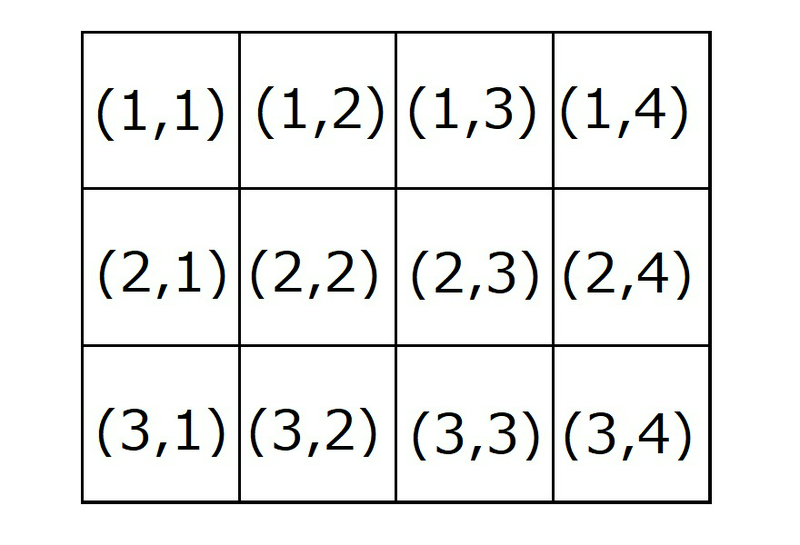﻿ ABC250 A - Adjacent Squares - Atcoder

# Home

Score : $100$ points

### Problem Statement

There is a grid with $H$ horizontal rows and $W$ vertical columns. Let $(i,j)$ denote the square at the $i$-th row from the top and the $j$-th column from the left.
Find the number of squares that share a side with Square $(R, C)$.

Here, two squares $(a,b)$ and $(c,d)$ are said to share a side if and only if $|a-c|+|b-d|=1$ (where $|x|$ denotes the absolute value of $x$).

### Constraints

• All values in input are integers.
• $1 \le R \le H \le 10$
• $1 \le C \le W \le 10$

### Input

Input is given from Standard Input in the following format:

$H$ $W$
$R$ $C$


### Output

Print the answer as an integer.

### Sample Input 1

3 4
2 2


### Sample Output 1

4


We will describe Sample Inputs/Outputs $1,2$, and $3$ at once below Sample Output $3$.

### Sample Input 2

3 4
1 3


### Sample Output 2

3


### Sample Input 3

3 4
3 4


### Sample Output 3

2


When $H=3$ and $W=4$, the grid looks as follows.

• For Sample Input $1$, there are $4$ squares adjacent to Square $(2,2)$.
• For Sample Input $2$, there are $3$ squares adjacent to Square $(1,3)$.
• For Sample Input $3$, there are $2$ squares adjacent to Square $(3,4)$.### Sample Input 4

1 10
1 5


### Sample Output 4

2


### Sample Input 5

8 1
8 1


### Sample Output 5

1


### Sample Input 6

1 1
1 1


### Sample Output 6

0